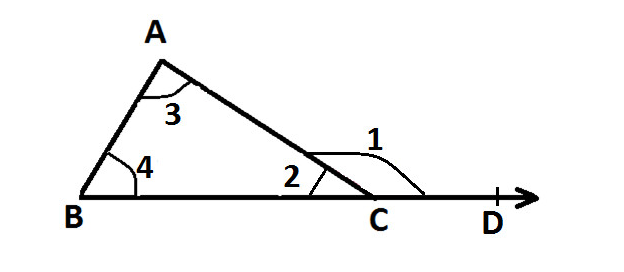Home/Class 7/Maths/

QuestionMathsClass 7

State whether the statements are True or False: The measure of any exterior angle of a triangle is equal to the sum of the measures of its two interior opposite angles.___

4.64.6Solution

Let a $$\Delta ABC$$ be a given triangle such that side $$BC$$ is extended to $$D$$, as shown in the figure below:Now, $$BCD$$ is a straight line, so, $$\angle 2 \;\&\;\angle 1$$ are linear pair.
$$\implies \angle 1+\angle 2=180^{\circ}...(1)$$
Now, we know that the sum of interior angles of a triangle is $$180^{\circ}$$.
In $$\Delta ABC$$, we have,
$$\implies \angle 2+\angle 3+\angle 4=180^{\circ}...(2)$$
Using $$(1)$$ and $$(2)$$,
$$\implies \angle 1+\angle 2=\angle 2+\angle 3+\angle 4$$
$$\implies \angle 1=\angle 3+\angle 4$$
Now, $$\angle 1$$ is an exterior angle and $$\angle 3,\angle 4$$are interior angles of $$\Delta ABC$$.
Therefore, it proves that the measure of any exterior angle of a triangle is equal to the sum of the measures of its two interior opposite angles.
Hence, the given statement is true.